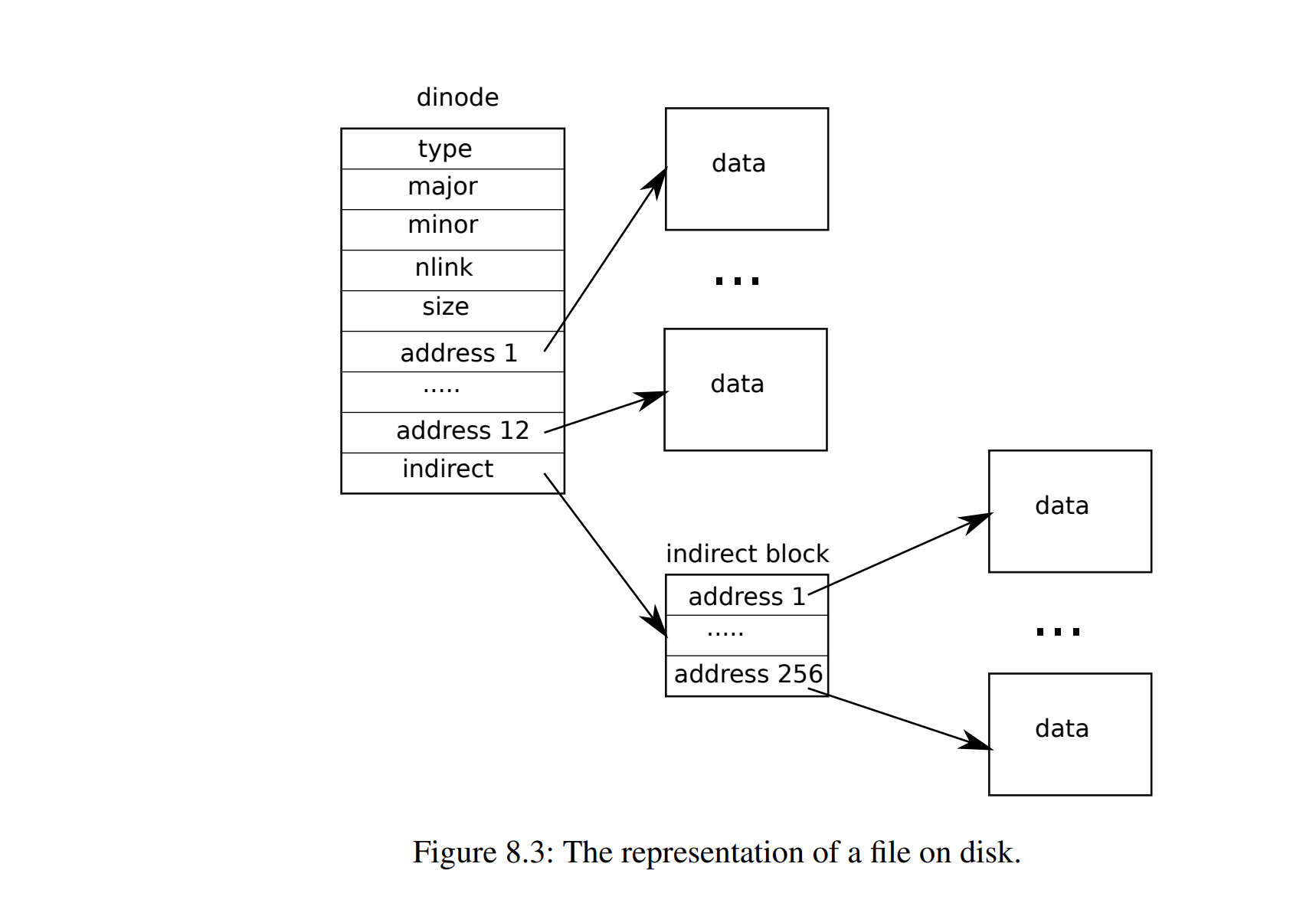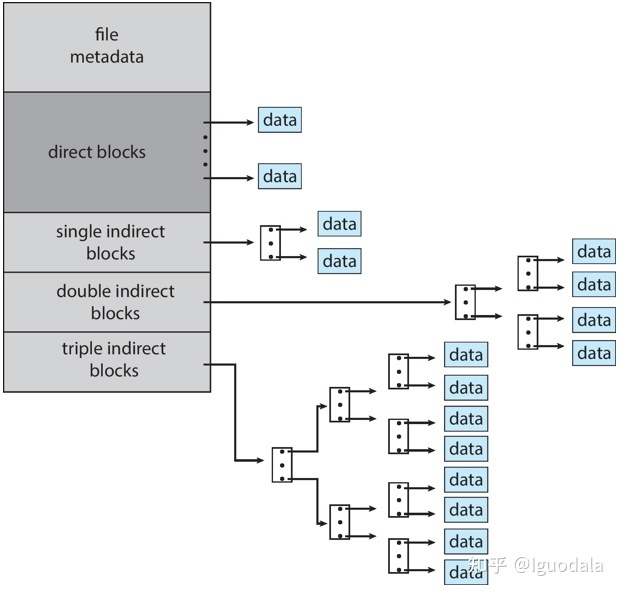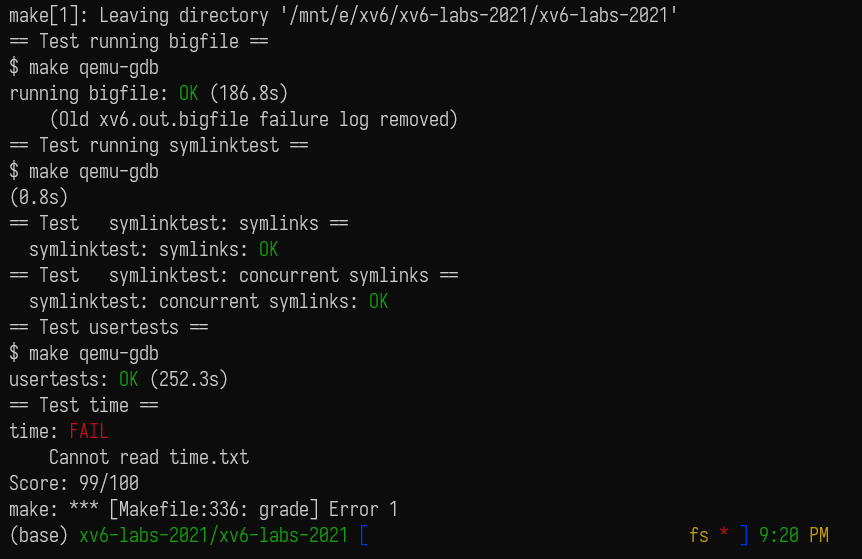upd@2022/9/14：最近把实验的代码放到 github 上了，如果需要参考可以查看这里：

https://github.com/ttzytt/xv6-riscv

# Lab10: file system

## Large files

### 描述

struct dinode {
short type;           // File type
short major;          // Major device number (T_DEVICE only)
short minor;          // Minor device number (T_DEVICE only)
uint size;            // Size of file (bytes)
};### 代码

#define NDIRECT 11 // 减少一个直接索引，增加一个二级间接索引
#define NINDIRECT (BSIZE / sizeof(uint))
#define NBI_INDIRECT NINDIRECT * NINDIRECT // 二级间接索引提供的块
#define MAXFILE (NDIRECT + NINDIRECT + NBI_INDIRECT) // 

//in fs.h
// On-disk inode structure
struct dinode {
short type;           // File type
short major;          // Major device number (T_DEVICE only)
short minor;          // Minor device number (T_DEVICE only)
uint size;            // Size of file (bytes)
};
// in file.h
// in-memory copy of an inode
struct inode {
uint dev;           // Device number
uint inum;          // Inode number
int ref;            // Reference count
struct sleeplock lock; // protects everything below here
int valid;          // inode has been read from disk?

short type;         // copy of disk inode
short major;
short minor;
uint size;
};

bmap()

// in fs.c
……
bn -= NINDIRECT;
// bn 代表还剩多少个

if(bn < NBI_INDIRECT){
a = (uint *)bp->data;

uint idx_b1 = bn / NINDIRECT; // 取得 bn 对应的，一级间接块在 addr 中的下标
if((addr = a[idx_b1]) == 0){  // 一个一级块负责 256 个二级块，这里检测对应一级块是否存在
log_write(bp);
// 标志这个块被修改了，随后会更新到磁盘的日志区
// 修改是因为，我们给这个储存块指针的块添加了一个新的块指针
}

brelse(bp); // 释放块缓存

a = (uint *)bp2->data;
uint idx_b2 = bn % NINDIRECT;
log_write(bp2);
}
brelse(bp2);
}
……

itrunc()

bmap() 相同，很多地方可以参考一级间接索引的实现。主要的思路类似递归，先遍历每个一级块，检查里面是否有数据，如果有，就去遍历这个一级块里的二级块。

// in fs.c
……
if(ip->addrs[NDIRECT + 1]){ // 判断 inode 是否使用了二级间接索引
a = (uint*)bp->data;
for (i = 0; i < NINDIRECT; i++){ // 遍历一级块
if(a[i]){ // 如果有数据，就遍历这个一级块里的二级块
struct buf* bp2 = bread(ip->dev, a[i]); // 获取这个块的对应缓存
uint *a2 = bp2->data;
for(j = 0; j < NINDIRECT; j++){
if(a2[j])
bfree(ip->dev, a2[j]); // a2[j] 存的是块号，这里把磁盘中这个块的内容清空了。或者说释放
}

brelse(bp2); // 释放块缓存
bfree(ip->dev, a[i]); // 释放磁盘中的块
// 和 a[i] 对应的是 bp2
// a[i] 是块号，bp2 是实际的块缓存
}
}
brelse(bp); // 释放缓存
bfree(ip->dev, ip->addrs[NDIRECT + 1]); // 释放磁盘块
}
……

### 思路

//in fcntl.h
#define O_RDONLY  0x000
#define O_WRONLY  0x001
#define O_RDWR    0x002
#define O_CREATE  0x200
#define O_TRUNC   0x400
#define O_NOFOLLOW 0x800

//in stat.h
#define T_DIR     1   // Directory
#define T_FILE    2   // File
#define T_DEVICE  3   // Device
#define T_SYMLINK 4   // 软连接

### 代码

sys_symlink()

uint64 sys_symlink(){
char tar_path[MAXPATH], path[MAXPATH];
try(argstr(0, tar_path, MAXPATH), return -1);
try(argstr(1, path, MAXPATH), return -1);
struct inode* ip;

begin_op();
ip = create(path, T_SYMLINK, 0, 0); // 创建一个文件，返回其 inode（因为没注释，我其实不是很确定这个函数
// 的用法，只是根据其实现猜测的）
if(ip == 0){
end_op();
return -1;
}
try(writei(ip, 0, tar_path, 0, strlen(tar_path)), end_op(); return -1);
// writei 其实就是往某个 inode 中写数据，这里把软链接想要指向的路径写进去了
iunlockput(ip);
// 使用完 inode 后的标准操作
// 先释放了锁，然后释放这个 inode
// 这里对于 inode 的 iput() 和对于块缓存的 brelse() 很相似
// 都是先减少了引用计数，然后判断是否可用真正的释放
end_op();
return 0;
}

sys_open()

\\ in sysfile.c
if(omode & O_CREATE){
ip = create(path, T_FILE, 0, 0);
if(ip == 0){
end_op();
return -1;
}
} else {
if((ip = namei(path)) == 0){
end_op();
return -1;
}
ilock(ip);
if(ip->type == T_DIR && omode != O_RDONLY){
iunlockput(ip);
end_op();
return -1;
}
}

\\ in sysfile.c
if(!(omode & O_NOFOLLOW)){
int rec_left = 10; // 递归次数限制，软链接可能成环
struct inode* next_file;

if(readi(ip, 0, path, 0, MAXPATH) == 0){
iunlockput(ip);
end_op();
return -1;
}

if((next_file = namei(path)) == 0){
// namei 可用从一个路径获得 inode
iunlockput(ip);
end_op();
return -1;
}
iunlockput(ip); // 储存链接的文件已经使用完了
ip = next_file;
rec_left--;
ilock(ip); // 在这里加锁而不在 while 的下面是因为如果这个 inode 不是一个软链接
// 我们还是需要持有这个锁的，因为后面的处理代码会修改 inode
}
if(rec_left <= 0){
iunlockput(ip);
end_op();
return -1;
}
}

// Lock the given inode.
// Reads the inode from disk if necessary.
void
ilock(struct inode *ip)
{
struct buf *bp;
struct dinode *dip;

if(ip == 0 || ip->ref < 1)
panic("ilock");

acquiresleep(&ip->lock);

if(ip->valid == 0){
dip = (struct dinode*)bp->data + ip->inum%IPB;
ip->type = dip->type;
ip->major = dip->major;
ip->minor = dip->minor;
ip->size = dip->size;
brelse(bp);
ip->valid = 1;
if(ip->type == 0)
panic("ilock: no type");
}
}## 总结

r = symlink("/testsymlink/4", "/testsymlink/3");

close(fd1);
close(fd2); // 问题

fd1 = open("/testsymlink/4", O_CREATE | O_RDWR);
if(fd1<0) fail("Failed to create 4\n");

sys_close() -> fileclose() -> iput() -> itrunc() -> bread()：

static void
bfree(int dev, uint b)
{
struct buf *bp;
int bi, m;

bi = b % BPB;
m = 1 << (bi % 8);
if((bp->data[bi/8] & m) == 0)
panic("freeing free block"); // 这里
bp->data[bi/8] &= ~m;
log_write(bp);
brelse(bp);
}

struct inode {
uint dev;           // Device number
uint inum;          // Inode number
int ref;            // Reference count
struct sleeplock lock; // protects everything below here
int valid;          // inode has been read from disk?

short type;         // copy of disk inode
short major;
short minor;
};